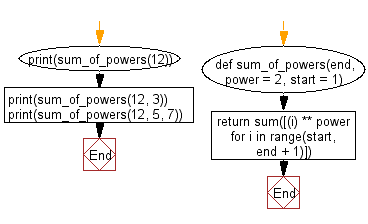﻿ Python Math: Get the sum of the powers of all the numbers from start to end (both inclusive) - w3resource# Python Math: Get the sum of the powers of all the numbers from start to end (both inclusive)

## Python Math: Exercise-85 with Solution

Write a Python program to get the sum of the powers of all the numbers from start to end (both inclusive).

• Use range() in combination with a list comprehension to create a list of elements in the desired range raised to the given power.
• Use sum() to add the values together.
• Omit the second argument, power, to use a default power of 2.
• Omit the third argument, start, to use a default starting value of 1.

Sample Solution:

Python Code:

``````def sum_of_powers(end, power = 2, start = 1):
return sum([(i) ** power for i in range(start, end + 1)])

print(sum_of_powers(12))
print(sum_of_powers(12, 3))
print(sum_of_powers(12, 5, 7))
```
```

Sample Output:

```650
6084
618507
```

Flowchart:## Visualize Python code execution:

The following tool visualize what the computer is doing step-by-step as it executes the said program:

Python Code Editor:

Have another way to solve this solution? Contribute your code (and comments) through Disqus.

What is the difficulty level of this exercise?

Test your Programming skills with w3resource's quiz.

﻿

```>>> students = [{'name': 'John', 'score': 98}, {'name': 'Mike', 'score': 94}, {'name': 'Jennifer', 'score': 99}]